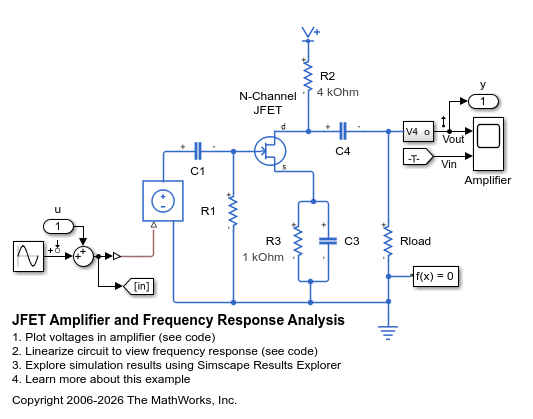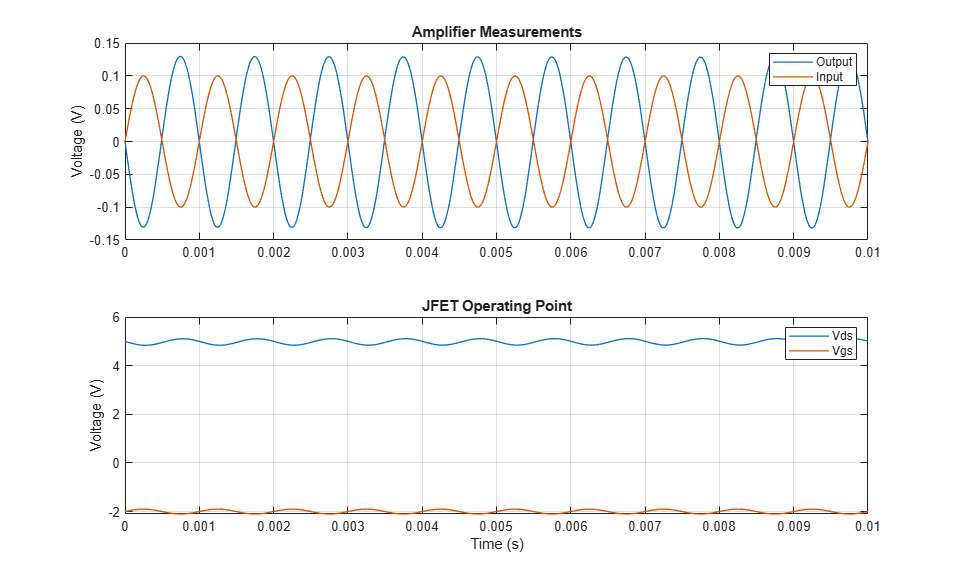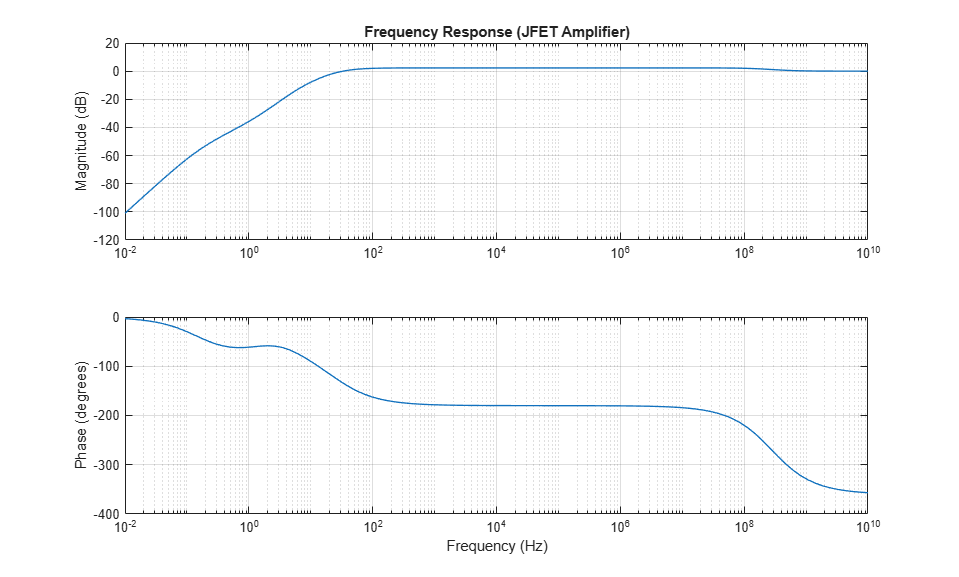# JFET Amplifier and Frequency Response Analysis

This example shows an audio amplifier circuit based on an N-channel JFET. The desired operating point is taken to be Vds=5V, Id=2mA and Vgs=-2V. The manufacturer datasheet gives the JFET forward transfer conductance and output conductance values as 3mS and 50uS. These values are used to populate the mask of the N-Channel JFET block.

The bias resistor values are calculated as follows:

Resistor R1 effectively ties the gate to ground. Hence the voltage across resistor R3 is -Vgs, and must be 2V. Hence R3=-Vgs/Id=2/2e-3 = 1K ohm.

The total voltage across R3, the JFET drain-source connections and R2 must be 15V. Hence the voltage across R2 is 8V if Vds is 5V, and R2=8/2e-3 = 4K ohm.

C3 has to be large enough such that at the lowest frequency of interest (20Hz), it is effectively a short circuit. C4 is chosen so that the loss in gain compared to the mid-band gain is about 6dB.

If you have Simulink® Control Design™, then to plot the frequency response, open the model JFETFrequencyResponse. On the Apps tab, under Control Systems, click Model Linearizer. In the Model Linearizer, on the Linear Analysis tab, in the Linearize section, click Bode. The linearization points were defined in this model by right-clicking on a Simulink line, and selecting Linearization Points.

### Model### Simulation Results from Simscape Logging

The plots below shows the voltage from the amplifier circuit. The upper plot shows the gain of the amplifier, and the lower plot shows that the JFET is being tested near its desired operating point.### Frequency Response### Results from Real-Time Simulation

This example has been tested on a Speedgoat Performance real-time target machine with an Intel® 3.5 GHz i7 multi-core CPU. This model can run in real time with a step size of 150 microseconds.